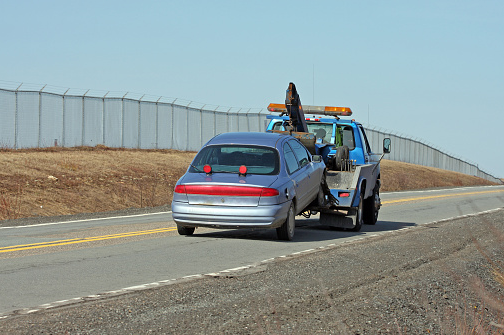## Towing a Car

A damaged 1200-kg car is being towed by a truck. Neglecting the friction, air drag, and rolling resistance, determine the extra power required (a) for constant velocity on a level road, (b) for constant velocity of 50 km/h on a 30 degree (from horizontal) uphill road, and (c) to accelerate on a level road from stop to 90 km/h in 12 seconds.Hint
The power due to potential energy:
$$\dot{W}_{g}=mg(z_{2}-z_{1})/\Delta t$$$where $$m$$ is the mass, $$g$$ is the acceleration due to gravity, $$\Delta z$$ is the change in height, and $$\Delta t$$ is the change in time. Hint 2 The power from kinetic energy: $$\dot{W}_{a}=\frac{1}{2}m(V_{2}^{2}-V_{1}^{2})/\Delta t$$$
where $$m$$ is the mass, $$V$$ is the velocity, and $$\Delta t$$ is the change in time.
The total power required for each case is the sum of the rates of changes in potential and kinetic energies. That is:
$$\dot{W}_{total}=\dot{W}_{a}+\dot{W}_{g}$$$where $$\dot{W}_a$$ is the power from kinetic energy, and $$\dot{W}_g$$ is the power from potential energy. (a) Zero. (b) $$\dot{W}_{a}=0$$ . Thus, $$\dot{W}_{total}=\dot{W}_{g}=mg(z_{2}-z_{1})/\Delta t=mg\frac{\Delta z}{\Delta t}=mgV_{2}=mgVsin(30^{\circ})$$$
where $$m$$ is the mass, $$g$$ is the acceleration due to gravity, $$\Delta z$$ is the change in height, $$\Delta t$$ is the change in time, $$V$$ is the velocity. Thus, the extra power on an upward hill is:
$$=(1200kg)(9.81m/s^2)(\frac{50,000m}{3600s})(\frac{1kJ/kg}{1000m^2/s^2})(0.5)=81.7\:kW$$$(c) $$\dot{W}_{g}=0$$ . Thus, $$\dot{W}_{total}=\dot{W}_{a}=\frac{1}{2}m(V_{2}^{2}-V_{1}^{2})/\Delta t$$$
where $$m$$ is the mass, $$V$$ is the velocity, and $$\Delta t$$ is the change in time. Thus, the extra power to accelerate from rest is:
$$=\frac{1}{2}(1200kg)[(\frac{90,000m}{3600s})^{2}-0](\frac{1kJ/kg}{1000m^2/s^2})/(12s)=31.3\:kW$$\$
(a) Zero
(b) 81.7 kW
(c) 31.3 kW